Color

Background

Done

# Visualizing Recursion with the Sierpinski Triangle

by kirupa   |   filed under Data Structures and Algorithms

To re-use a joke you've probably seen a bunch of times, recursion is the rare gift that keeps on giving, and giving, and...giving! To better understand how recursion works, beyond watching monks move some disks from one place to another, we are going to look at a very visual example. We are going to look into how the famous Sierpinksi triangle is generated.

Onwards!

## Meet the Sierpinksi Triangle

Cutting right to the chase, the Sierpinski triangle looks as follows (minus the animals):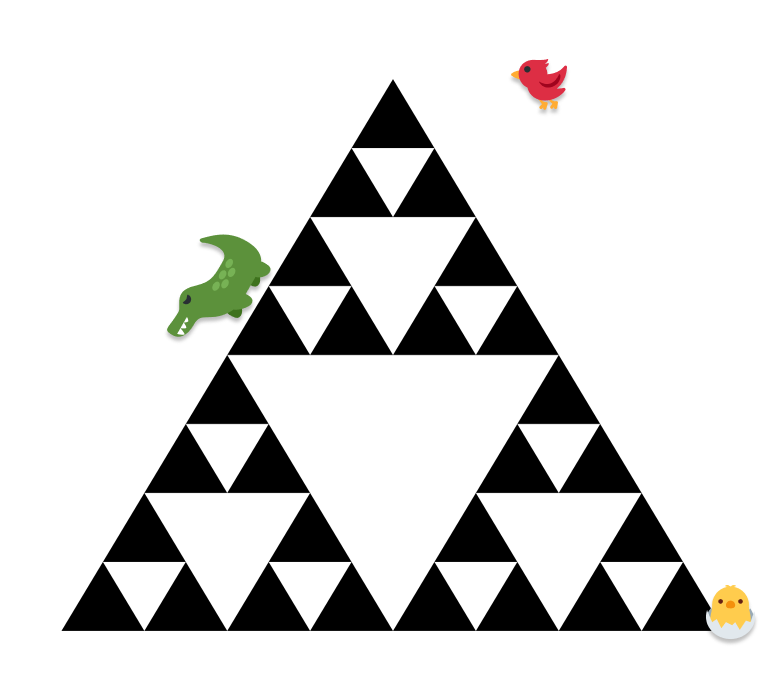If we take a few steps back, the Sierpinski triangle belongs to a family of visuals known as a fractal. A fractal is a never-ending pattern that repeats itself at smaller and smaller scales. Like many fractals, the Sierpinski triangle is generated, and the more time (aka iterations) we spend generating our Sierpinski triangle, the more detailed it will look.

Starting at the very beginning, let's start with our 0th iteration, where we will have the least amount of detail. In this low-detail world, our amazing Sierpinski triangle will be nothing more than a run-of-the-mill equilateral triangle:With each increasing iteration, our Sierpinski triangle will get more detailed, with more triangles making an appearance. At the 1st iteration, our single triangle turns into three dark triangles:This three-triangle variation is famous for many things, but it is well known to a bunch of us video gamers as the Triforce in the Zelda series of video games (original image source):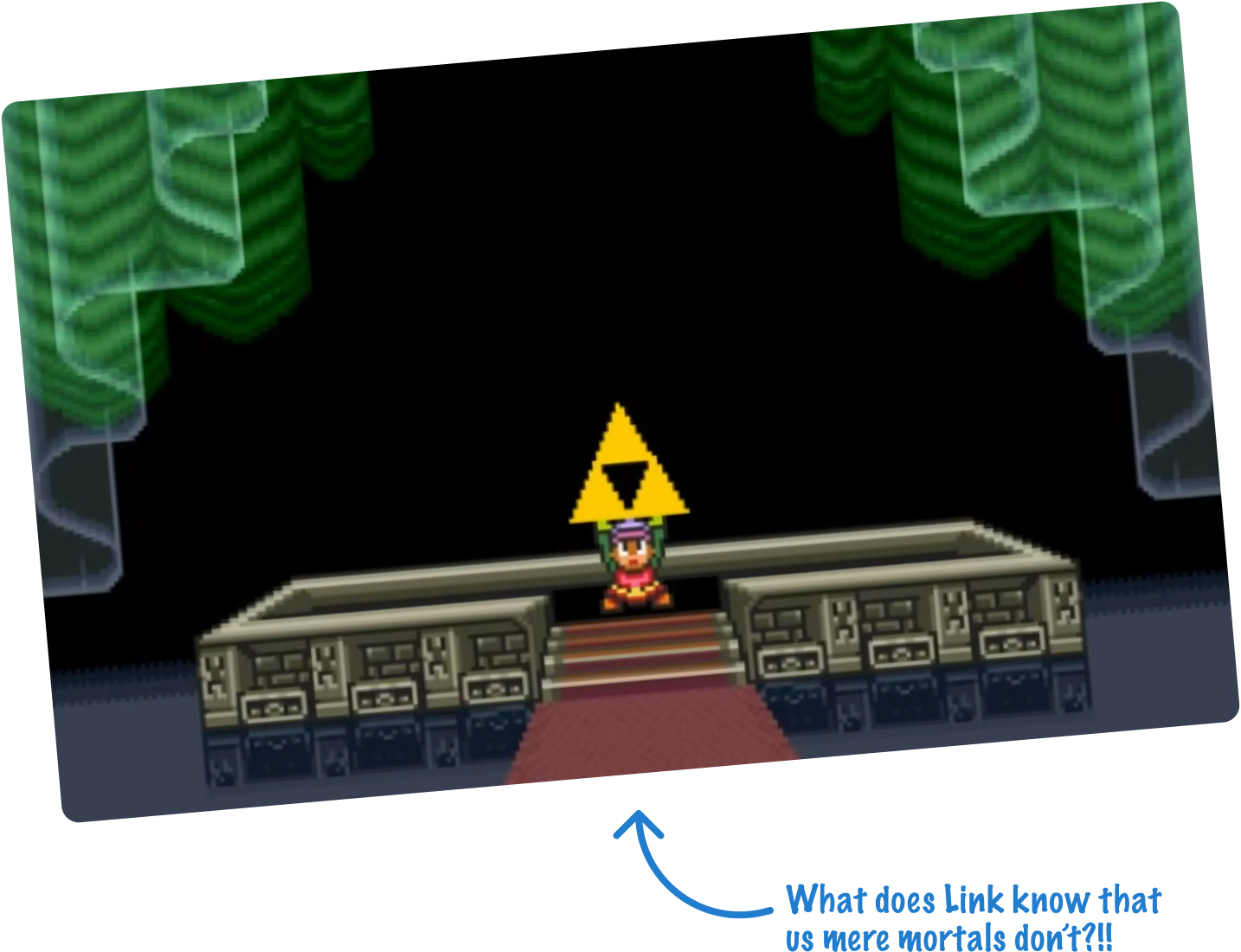Anyway, I digress. With each iteration, we'll find that more triangles appear. At the 2nd iteration, each of the darker triangles is further divided into more individual triangles: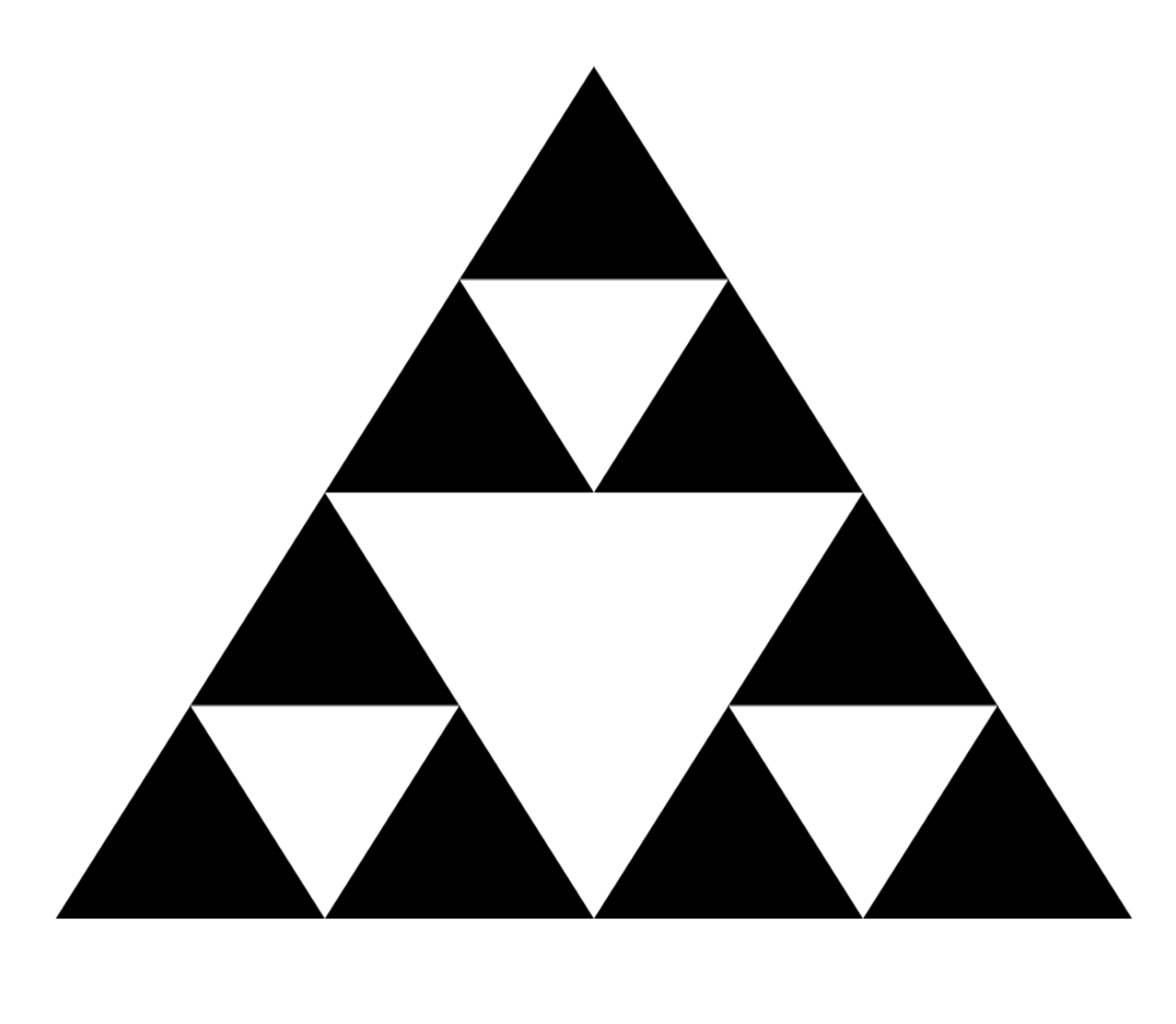There is a pattern to how these triangles appear. Inside each black triangle, the midpoints of each edge create the edges that will divide the larger triangle into three smaller triangles: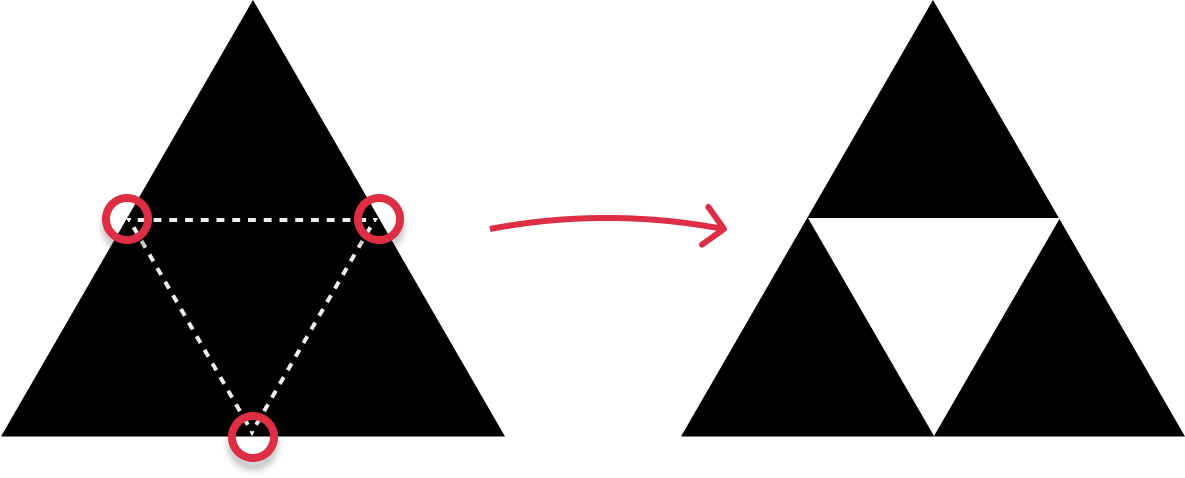This pattern will repeat forever, where, with each increasing iteration, every black triangle will be divided further into three more black triangles. The interesting detail is this. The approach we will be using to create smaller triangles out of a larger triangle revolves fully around our old friend recursion. More on that momentarily.

Continuing to go deeper into our iterations, at our 3rd iteration, our Sierpinski triangle starts to look a lot more detailed:Further emphasizing that this pattern will repeat forever, let's jump ahead a few iterations to the 6th iteration. What we see will look as follows:This Sierpinski triangle looks pretty detailed. There is no limit to how many iterations we want to go through. We can theoretically go forever, provided we have the computational power to draw many trianges or have the display capabilities to render ever-smaller pixels in a visible way. For now, we'll stop at the 6th iteration. This level of detail is great for what we are trying to do.

## The Recursive Algorithm and Implementation

The recursive approach to generate a Sierpinski triangle involves breaking down a larger equilateral triangle into smaller equilateral triangles of equal size. Here's how the algorithm works:

1. Base Case: The base case of the recursion is when it reaches our specified iteration value
2. Divide: To create the Sierpinski triangle, we start with a single equilateral triangle. We then divide this triangle into smaller equilateral triangles by drawing a line between the midpoint of all three edges: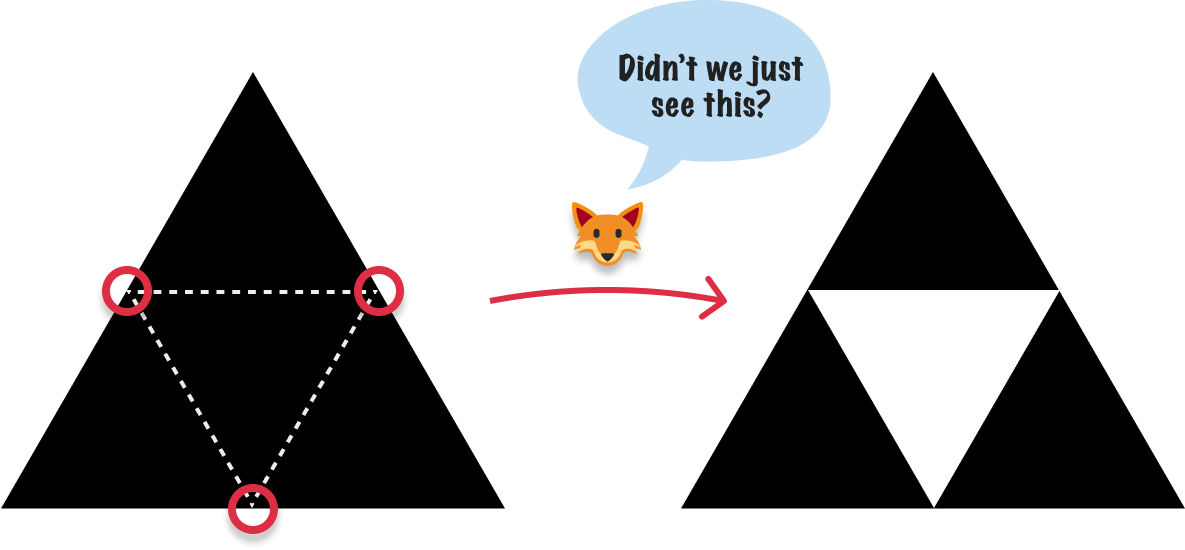1. Recurse: Now, we have three smaller triangles: the top one and the two triangles on the bottom corners. Apply the same algorithm recursively to each of these smaller triangles. This means dividing each of these smaller triangles into even smaller triangles and repeating this process until we reach the base case.
2. Draw: As we reach the base case, we stop dividing and start drawing the individual triangles. We'll draw the triangles at each step, creating a pattern that resembles the Sierpinski triangle.

If we turn all of these words into code, here is what the algorithm will look like:

``````// Function to draw a triangle
function drawTriangle(x1, y1, x2, y2, x3, y3) {
// Set the fill color for the triangles
ctx.fillStyle = 'black';

ctx.beginPath();
ctx.moveTo(x1, y1);
ctx.lineTo(x2, y2);
ctx.lineTo(x3, y3);
ctx.closePath();
ctx.fill();
}

// Recursive function to generate Sierpinski triangle
function sierpinski(x1, y1, x2, y2, x3, y3, iterations) {
if (iterations === 0) {
drawTriangle(x1, y1, x2, y2, x3, y3);
} else {
const mid1x = (x1 + x2) / 2;
const mid1y = (y1 + y2) / 2;
const mid2x = (x2 + x3) / 2;
const mid2y = (y2 + y3) / 2;
const mid3x = (x1 + x3) / 2;
const mid3y = (y1 + y3) / 2;

sierpinski(x1, y1, mid1x, mid1y, mid3x, mid3y, iterations - 1);
sierpinski(mid1x, mid1y, x2, y2, mid2x, mid2y, iterations - 1);
sierpinski(mid3x, mid3y, mid2x, mid2y, x3, y3, iterations - 1);
}
}``````

If we put this code as part of a full end-to-end example, this is what the full HTML, CSS, and JavaScript will look like:

``````<!DOCTYPE html>
<html>

<title>Sierpinski Triangle Visualization</title>
<style>
body {
margin: 0;
overflow: hidden;
}

canvas {
display: block;
width: 690px;
height: 600px;
transform: scale(.5);
}
</style>

<body>
<canvas id="canvas"></canvas>

<script>
const canvas = document.getElementById('canvas');
const ctx = canvas.getContext('2d');

// Function to draw a triangle
function drawTriangle(x1, y1, x2, y2, x3, y3) {
// Set the fill color for the triangles
ctx.fillStyle = 'black';

ctx.beginPath();
ctx.moveTo(x1, y1);
ctx.lineTo(x2, y2);
ctx.lineTo(x3, y3);
ctx.closePath();
ctx.fill();
}

// Recursive function to generate Sierpinski triangle
function sierpinski(x1, y1, x2, y2, x3, y3, iterations) {
if (iterations === 0) {
drawTriangle(x1, y1, x2, y2, x3, y3);
} else {
const mid1x = (x1 + x2) / 2;
const mid1y = (y1 + y2) / 2;
const mid2x = (x2 + x3) / 2;
const mid2y = (y2 + y3) / 2;
const mid3x = (x1 + x3) / 2;
const mid3y = (y1 + y3) / 2;

sierpinski(x1, y1, mid1x, mid1y, mid3x, mid3y, iterations - 1);
sierpinski(mid1x, mid1y, x2, y2, mid2x, mid2y, iterations - 1);
sierpinski(mid3x, mid3y, mid2x, mid2y, x3, y3, iterations - 1);
}
}

// Set canvas size to match the window size
canvas.width = window.innerWidth;
canvas.height = window.innerHeight;

// Define the initial triangle points
const x1 = canvas.width / 2;
const y1 = 50;
const x2 = 50;
const y2 = canvas.height - 50;
const x3 = canvas.width - 50;
const y3 = canvas.height - 50;

// Set the iterations of the recursion (increase for more detail)
const iterations = 5;

// Draw the Sierpinski triangle
sierpinski(x1, y1, x2, y2, x3, y3, iterations);
</script>
</body>

</html>``````

This markup and code contains what we need for both generating the many triangles as well as displaying them on the Canvas. When we preview this in our browsers, we'll see a fairly detailed Sierpinksi triangle displayed:

To adjust the level of detail, change the value of the iterations variable in our code. The higher the iterations value, the more detailed our Sierpinski triangle will be.

## Calculating the Number of Triangles

We can quickly see that our Sierpinski triangle is made up of many MANY smaller triangles. Exactly how many triangles are there? We can find out mathematically. Let's denote that the number of triangles in the Sierpinski triangle at the n-th iteration as T(n). The pattern can be described as follows:

Base Case: At the initial iteration (n = 0), there is one large equilateral triangle, so T(0) = 1.

Iteration n = 1: In the first iteration, we divide the large equilateral triangle into 3 smaller equilateral triangles. So, T(1) = 3.

Iteration n = 2: In the second iteration, each of the 3 smaller triangles from the previous iteration is divided into 3 even smaller triangles. This results in 3 * 3 = 9 triangles. So, T(2) = 9.

Iteration n = 3: In the third iteration, each of the 9 smaller triangles from the previous iteration is divided into 3 even smaller triangles. This results in 9 * 3 = 27 triangles. So, T(3) = 27.

The pattern continues where, at each iteration, the number of triangles is tripled. We can generalize it further and say that the patternis 3 raised to the power of the iteration number. Mathematically this can be simplified as T(n) = 3n, which is the formula for calculating the number of triangles in our Sierpinski triangle.

## Conclusion

Almost any of the famous fractal shapes would have been good candidates to highlight how we can visualize recursion, but none are as simple and elegant like the Sierpinski triangle. We start with one triangle, cut out smaller ones from its corners, and keep doing this over and over. The more we keep dividing, the more triangles appear. The end result is a cool pattern that goes on forever. Pretty neat, right?

Just a final word before we wrap up. If you have a question and/or want to be part of a friendly, collaborative community of over 220k other developers like yourself, post on the forums for a quick response!